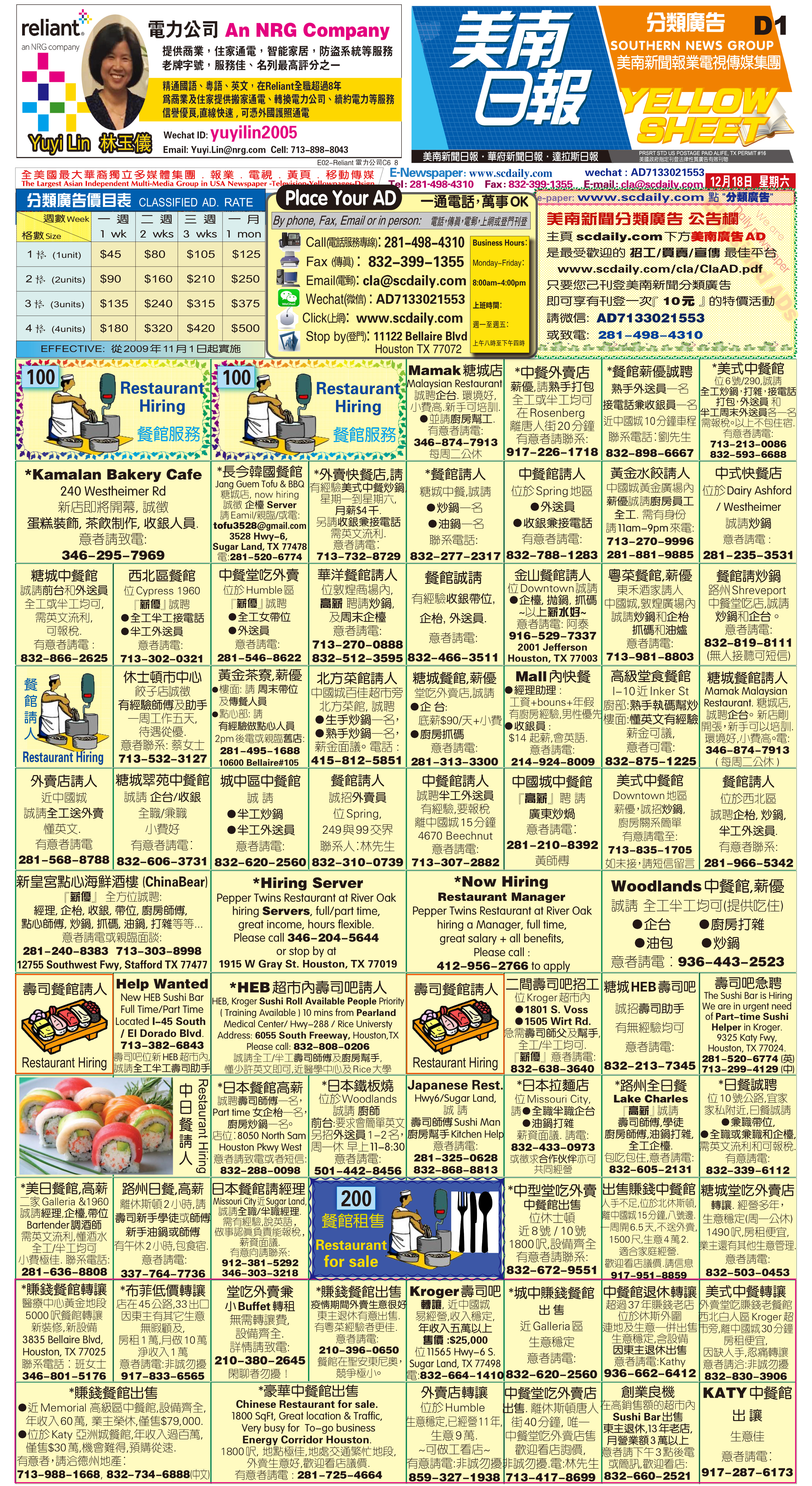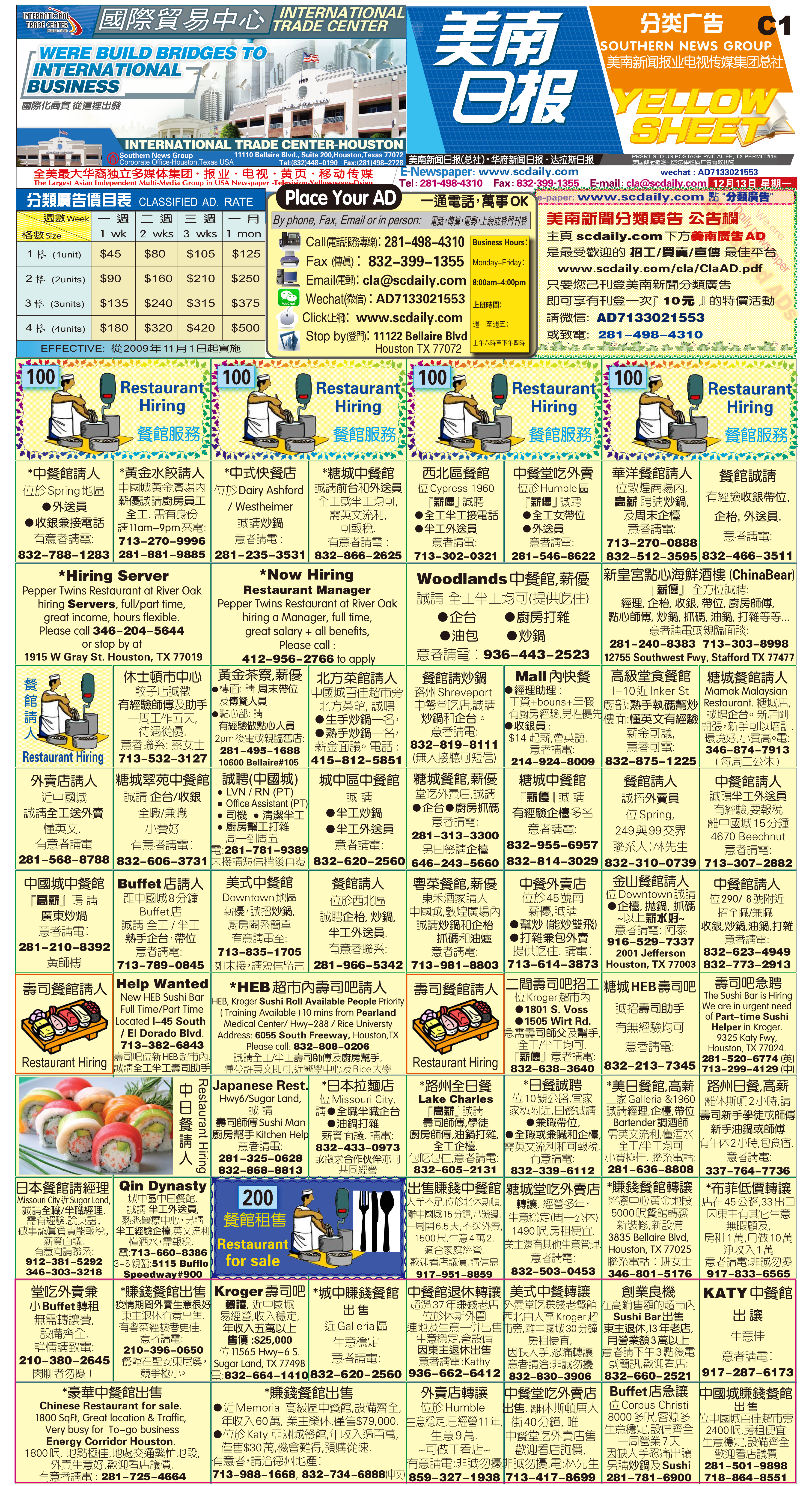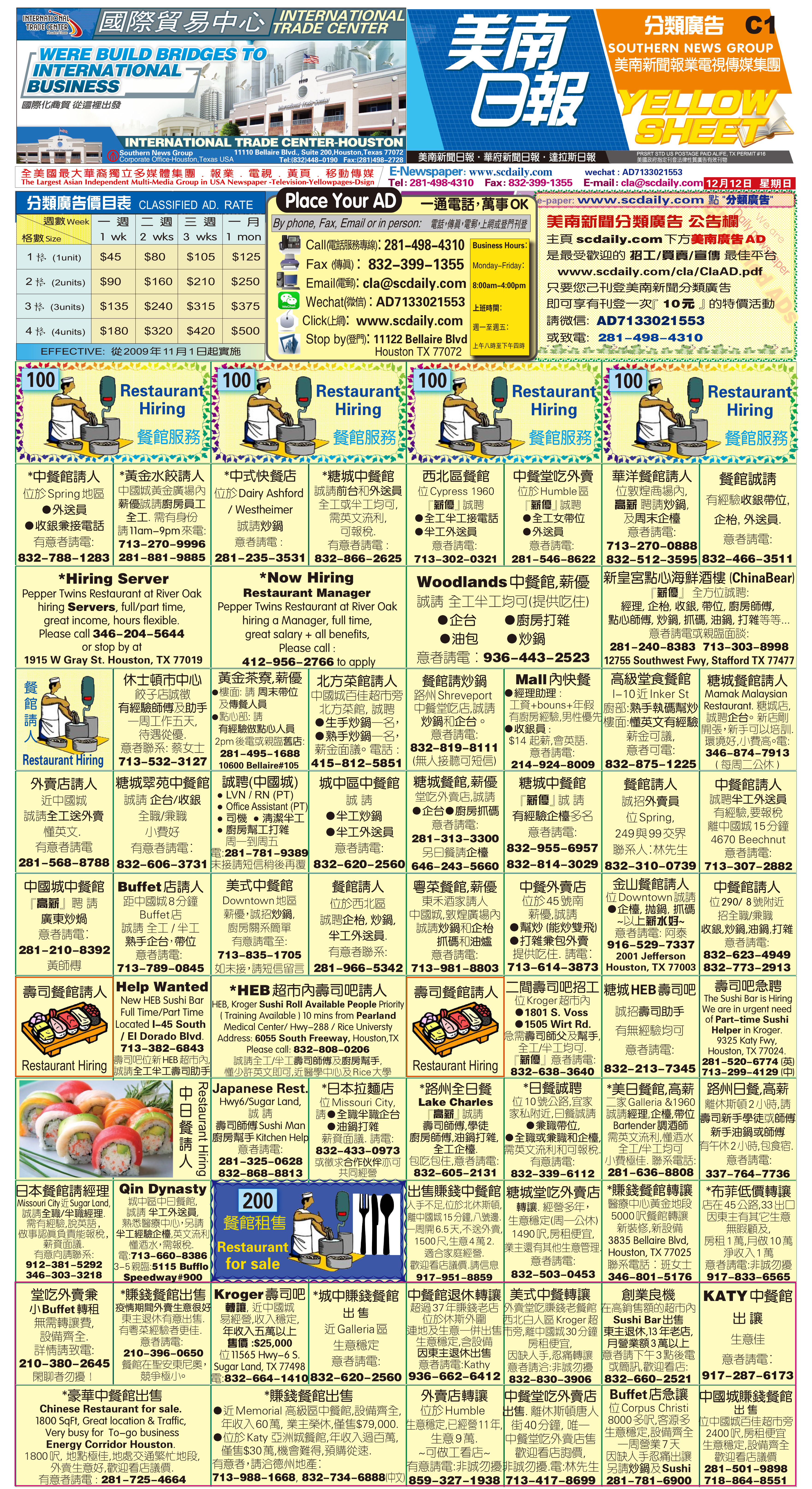211222 Epaper

 A Section B SectionC Section D Section211221 Epaper

 A Section B SectionC Section D Section211220 Epaper

 A Section B SectionC Section D Section211219 Epaper

 A Section B SectionC Section D Section211218 Epaper

 A Section B SectionC Section D Section211217 Epaper

 A Section B SectionC Section D Section211216 Epaper

 A Section B SectionC Section D Section211215 Epaper

 A Section B SectionC Section D Section211213 Epaper

 A Section B SectionC Section D Section211214 Epaper

 A Section B SectionC Section D Section211212 Epaper

 A Section B SectionC Section D Section